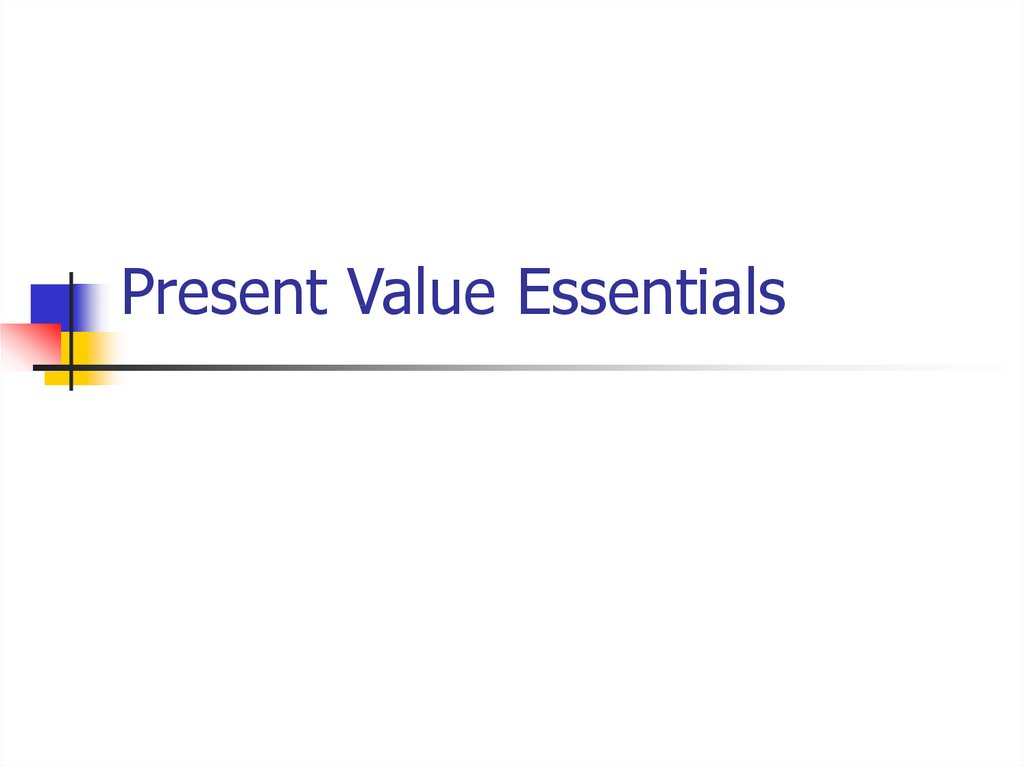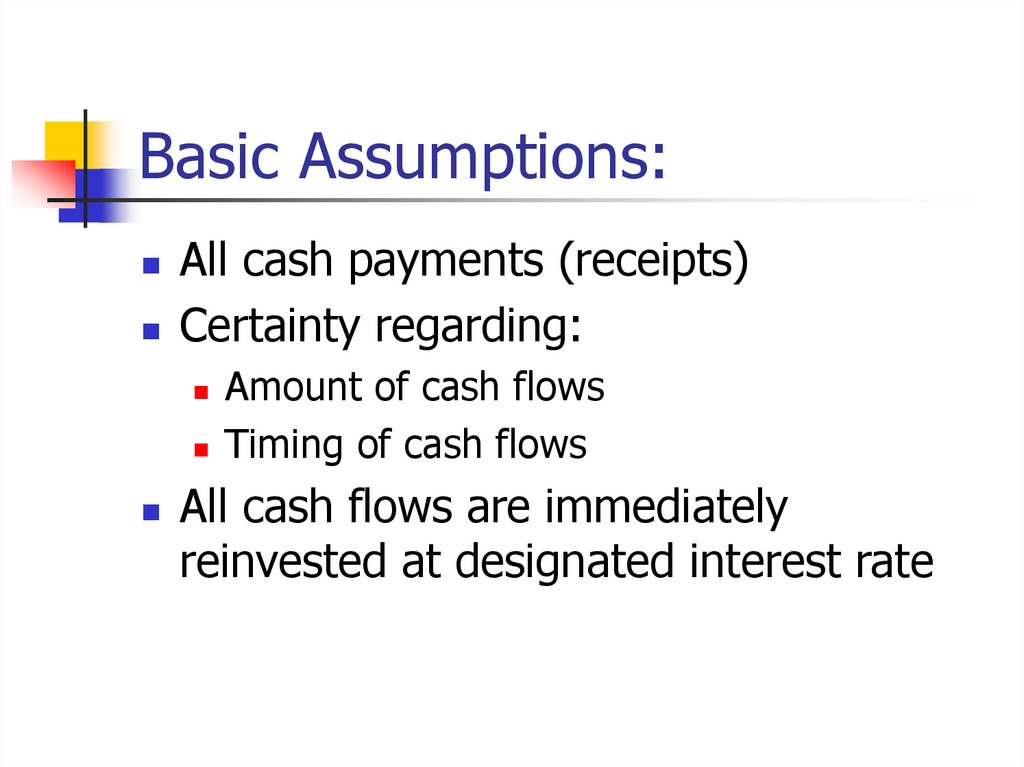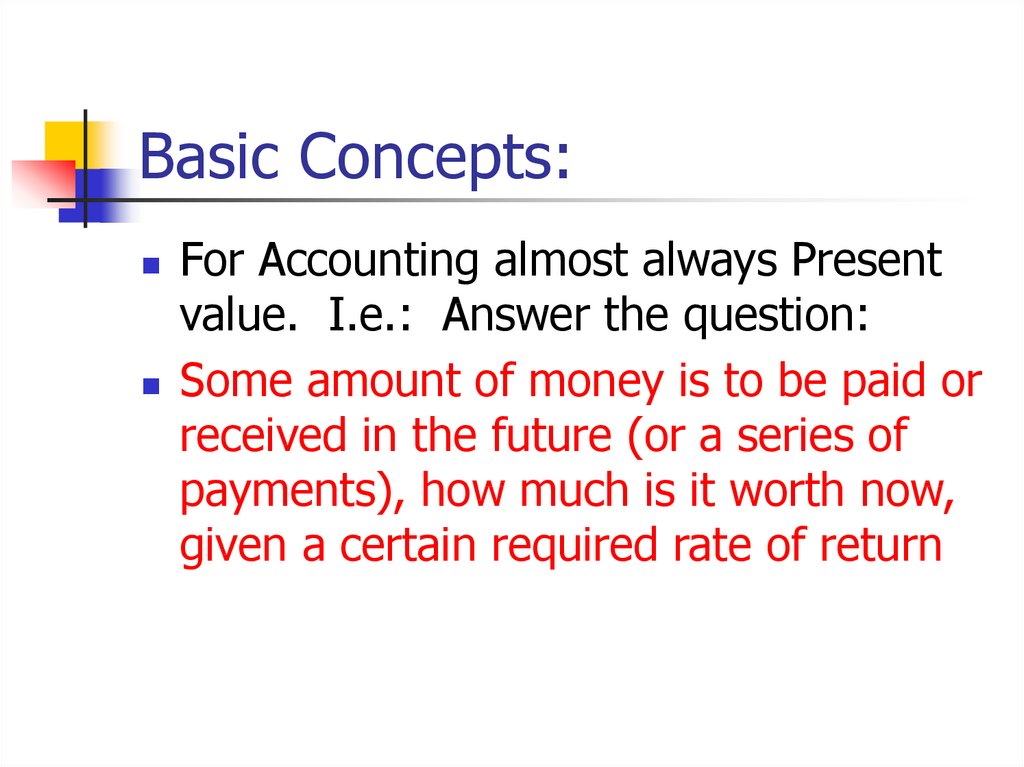# Present value essentials

## 2. Basic Assumptions:

All cash payments (receipts)
Certainty regarding:
Amount of cash flows
Timing of cash flows
All cash flows are immediately
reinvested at designated interest rate

## 3. Basic Concepts:

For Accounting almost always Present
Some amount of money is to be paid or
received in the future (or a series of
payments), how much is it worth now,
given a certain required rate of return

## 4. Basic Concepts I:

Time Value of Money:
Invested money earns interest (if in bank)
or some rate of return (if invested in
something else)
Compound interest:
Money earned on investment is reinvested
immediately at required rate of return

## 5. Basic Concepts II:

Interest; rate of return; discount rate:
For PV analysis they mean the same. From
now, only “interest” will be used
Future Value:
Value of an investment after a designated
period of time, given a specified interest
rate

## 6. Present Value vs. Future Value

Present value is based on future value,
specifically the compound interest
formula. Therefore
understand present value

## 7. Basic Future Value Concepts:

Invested money earns more money
\$1,000 today is worth more than
\$1,000 one year from today because:
\$1,000 invested at 10% grows to
\$1,100 in one year
\$1,100 is the future value of \$1,000 @
10% after one year

## 8. Future Value Example:

year 1
year 2
year 3
Value of investment
after three years:
Investment interest interest
rate
earned
\$100.00
10%
\$10.00
\$110.00
10%
\$11.00
\$121.00
10%
\$12.10
\$133.10

## 9. FV Example (alternate view):

FV Example
\$ 1,000 @ 10% grows to
(alternate view):
\$1,100 in one year
\$1,210 in two years
\$1,331 in three years OR
\$1,000 * 1.1*1.1*1.1 = \$1,331

## 10. Future Value Example:

Another way to determine the future value of \$100
invested to earn 10%, interest compounded
annually:Use the Compound interest formula:
(1 +r)n Where r = interest rate/compounding period
and n = number of compounding periods
(1 + .1)3 = 1.331 * 100 = \$133.10

## 11. Compounding:

Number of times per year interest is
calculated
May be annually, semi-annually,
quarterly, etc.
However: Interest rate is expressed
on annual basis, unless stated to be for
another period. Therefore: if annual
interest rate is 10% ----

## 12. Compounding:

Semi-annual: 5% twice a year
Quarterly: 2.5% four times a year
Monthly: 10/12% 12 times a year
In other words: If more than one
compounding period/year, interest rate
is divided by # of periods. # of years
multiplied by # of periods

## 13. Compounding:

Why does it matter? Because interest
10%, 3 years, semi-annual
compounding: (1 + .1/2)3*2 =
1.34 > (1 +.1)3 = 1.31

## 14. Future Value Calculation:

FV of r= 10%, annual compounding
and n= 3 years:
FV (r, n) = FV (10%,3) = 1.331
\$100 invested for 3 years at 10% =
\$100 * FV (10%, 3) = X
\$100 * 1.331 = X = \$133.10

## 15. Present Value (PV):

Accounting almost always wants to
know what something is worth now
3 years, how much is it worth today if
10% is the appropriate discount rate?
Use FV formula to answer the question:

## 16. PV of \$133.10 (to be paid or received in 3 years)

X * FV(10%,3)
= \$ 133.10
X * 1.331
= \$ 133.10
(X* 1.331)/1.331 = \$133.10/1.331 = \$100
PV = Reciprocal of FV OR 1/FV
therefore: PV(10%,3) = 1/FV(10%,3)
= 1/(1+.1)3 = .75132

## 17. PV of \$133.10 (to be paid or received in 3 years (again))

\$ 133.10 * PV(10%,3) = X
\$ 133.10 * .75132 = X = \$100
This is the equation you must use
Do not use the formula, use table

## 18. Part II Annuities

Basic PV used for single sum payments
E.g. a note payable due in 5 years
PV of Annuity used for questions
relating to a series of equal payments
at regular intervals
E.g. car payments, payments on a student
loan

## 19. PV of 3 payments of \$ 100 each?

Payments made at end of each of the
next three years, 10% interest rate:
PVA \$100 (10%,3)

## 20. PV annuity (PVA) \$100, 10%, 3 years:

Option 1:
we could express the above as follows:
PV
end of year 1 \$100.00 (10%,1)
0.9091 \$90.91
end of year 2 \$100.00 (10%,2)
0.8264 \$82.64
end of year 3 \$100.00 (10%,3)
0.7513 \$75.13
\$248.68

## 21. PV annuity (PVA) \$100, 10%, 3 years:

Option 2: Use simple algebra, factor out
constant:
Restated equation:
\$100 * (.9091 + .8264 + .7531) = X
\$100 * 2.4868
= X = \$248.68

## 22. PV annuity (PVA)

Present value of an annuity (PVA) 3 periods,
10% = (.9091 + .8264 + .7531) = 2.4868
Libby ordinary annuity table, page 748:
PVA (10%,3)
= 2.4869
Kimmel ordinary annuity table, Appendix C:
PVA (10%,3)
= 2.48685

## 23.

Present Value (PV) of \$ 1
period
1
2
3
1%
0.99
0.98
0.971
2%
0.98
0.961
0.942
10%
0.909
0.826
0.751
PV of an ordinary annuity of \$1
period
1
2
3
1%
0.99
1.97
2.941
2%
0.98
1.942
2.884
10%
0.909
1.736
2.487

## 24. PV annuity due (PVA due)

Difference: 1st payment is at beginning
of period compared to at the end for an
ordinary annuity
Example: Rent or lease payments
Libby does not have table for it
However: not a big problem

## 25. PVA due: 3 payments, 10%

Option 1:
we could express the above as follows:
beginning of year\$100.00
1
(10%,0)
1
\$100.00
beginning of year\$100.00
2
(10%,1)
0.9091
\$90.91
beginning of year\$100.00
3
(10%,2)
0.8264
\$82.64
\$273.55

## 26. PVA due: 3 payments, 10%

Option 2: Calculate the factor:
PVA due (10%,3)
= 1 +PVA(10%,2)
= 1 + 1.73554
= 2.73554 * \$ 100 = \$2.73.55
Compared to ordinary annuity: 2.4868